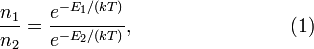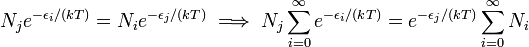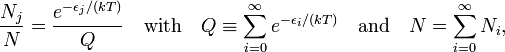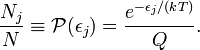# Boltzmann distribution

In classical statistical physics, the Boltzmann distribution expresses the relative probability that a subsystem of a physical system has a certain energy. The subsystem must part of a physical system that is in thermal equilibrium, that is, the system must have a well-defined (absolute) temperature. For instance, a subsystem can be a single molecule in, say, one mole of an ideal gas. Then the Boltzmann distribution applies to the energies of the individual gas molecules, provided the ideal gas is in thermal equilibrium.

The Boltzmann distribution (also known as the Maxwell-Boltzmann distribution) was proposed in 1859 by the Scotsman James Clerk Maxwell for the statistical distribution of the kinetic energies of ideal gas molecules. Consider an ideal gas of absolute temperature T in a vessel with volume V. Let n1 be the number of molecules in V with kinetic energy E1 and n2 be the number in V with kinetic energy E2, then according to the Maxwell-Boltzmann distribution law the relative probability is the ratio,$\frac{n_1}{n_2} = \frac{e^{-E_1/(kT)}}{e^{-E_2/(kT)}}, \qquad\qquad \qquad\qquad (1)$

where k is the Boltzmann constant. Most noticeable in this expression are (i) the energy in an exponential, (ii) the inverse temperature in the exponent, and (iii) the appearance of the natural constant k. Note that an argument of an exponential must be dimensionless and that accordingly kT has the dimension energy.

As discovered in 1868 by the Austrian Ludwig Boltzmann, the molecular energies in equation (1) may, in addition to the translational energy considered by Maxwell, contain interactions with an external field. Later, when it became evident that molecules possess different kinds of internal energy (rotation, vibration, electronic excitation, etc.), these energies were included, too, in the total energy E. If a system, as for instance a column of air of constant temperature (zero atmospheric lapse rate), is in the gravitational field of the Earth, each molecular energy contains the additional term mgh, where m is the molecular mass, g the gravitational acceleration and h the height of the molecule above the surface of the Earth. Thus, the ratio of the number density of molecules at height h1 and h2 with velocity v1 and v2, respectively, is given by the following Maxwell-Boltzmann distribution function,$\frac{n(h_1, v_1)}{n(h_2, v_1)} = \frac{e^{-(E_1 +mgh_1)/(kT)}}{e^{-(E_2 +mgh_2)/(kT)}} .$

The kinetic energies E1 and E2 are quadratic functions of the velocities. When we integrate the left- and right-hand side over all velocities in numerator and denominator we get on the right-hand side the same (finite) integrals in numerator and denominator that cancel each other. What remains is the barometric formula$\frac{n(h_1)}{n(h_2)} = \exp\Big[ - \frac{mg(h_1-h_2)}{kT}\Big],$

where n(h1) is the total (integrated over all velocities) number density of molecules at height h1 and n(h2) is the same at h2.

## Generalization

A few years after Boltzmann, the American Josiah Willard Gibbs gave a further formalization and generalization (ca. 1877). Gibbs introduced what he called an ensemble, a "supersystem" consisting of a statistically large number of identical systems. For instance, the systems may be identical vessels containing the same number of the same real gas molecules at the same temperature and pressure. Further Gibbs assumed that the ensemble, just like a system of gas molecules, is in thermal equilibrium, i.e., the systems are in thermal contact so that they can exchange heat. In the example that the systems are gas-filled vessels, this is achieved by requiring that the vessels are in mutual contact through heat-conducting walls.

Gibbs assumed that the Maxwell-Boltzmann law, equation (1), holds for the energies of the systems in the ensemble. This generalization, applied to the example of an ensemble consisting of gas-filled vessels, means that the energy of a gas molecule in equation (1) is replaced by the total energy of the molecules in a vessel; a "one-molecule" energy is promoted to a "one-vessel" energy. Because of molecular interactions (that are absent in an ideal gas), a one-vessel energy cannot be written as a sum of one-molecule energies. This is the main reason for Gibbs' generalization from a single vessel of gas to an ensemble of vessels, or, in more general terms, the generalization from an ideal gas to an ensemble of (rather arbitrary) systems. Just as the molecules in an ideal gas, the systems in the ensemble do not interact other than by exchanging heat.

The absolute probability for a system to have total energy εj can be obtained from equation (1) by normalizing. Let us assume, for convenience sake, that the one-system energies are discrete (as they often are in quantum mechanics) running from ε0 to ε , then$N_j e^{-\epsilon_i/(kT)} = N_i e^{-\epsilon_j/(kT)} \;\Longrightarrow\; N_j \sum_{i=0}^\infty e^{-\epsilon_i/(kT)} = e^{-\epsilon_j/(kT)} \sum_{i=0}^\infty N_i$

which gives$\frac{N_j}{N} = \frac{e^{-\epsilon_j/(kT)}}{Q} \quad \hbox{with} \quad Q \equiv \sum_{i=0}^\infty e^{-\epsilon_i/(kT)} \quad \hbox{and} \quad N =\sum_{i=0}^\infty N_i ,$

where Nj is the number of systems that have energy εj and N is the total number of systems in the ensemble (N must be very large—go to infinity—for statistics to apply). The ratio is a Boltzmann probability written as$\frac{N_j}{N} \equiv \mathcal{P}(\epsilon_j) = \frac{e^{-\epsilon_j/(kT)}}{Q}.$

The quantity Q is known as the partition function of the system. It is a sum over all energies that the system can have. In the older literature Q is called Zustandssumme, which is German for "sum over states", and is often denoted by Z. When we consider again the example of a vessel with interacting molecules at certain pressure and temperature, the sum in the partition function is over the possible total energies εi of all the molecules in the vessel.

In classical statistical physics, where energies are not discrete, the partition function of a system of n molecules is not a sum over the possible energies of the system, but an integral over the 6n-dimensional phase space (space of momenta and positions). In the framework of the "old quantum theory" it was discovered in 1912 that the classical partition function of n molecules must multiplied by a quantum factor. The classical partition function is,$Q_\mathrm{class} = \frac{1}{n! h^{3n}} \int e^{-\epsilon(p,q)/(kT)} \mathrm{d}\mathbf{q}_1 \cdots \mathrm{d}\mathbf{q}_n \,\mathrm{d}\mathbf{p}_1 \cdots \mathrm{d}\mathbf{p}_n,$

where h is Planck's constant and n! = 1 × 2× ... × n (the factorial of n).

As a final remark: in quantum statistical thermodynamics the Boltzmann distribution appears as the high-temperature limit of both Bose-Einstein statistics (valid for bosons) and Fermi-Dirac statistics (valid for fermions).

## Note

1. L. Boltzmann, Studien über das Gleichgewicht der lebendigen Kraft zwischen bewegten materiellen Punkten [Studies on the equilibrium of the kinetic energy of material points in motion], Sitzungsberichte der kaiserlichen Akademie der Wissenschaften Math.-naturw. Classe II., pp. 517–560, vol. 58 (1868)
2. D. ter Haar, Elements of Statistical Mechanics, Holt, Rinehart and Winston, New York (1961), p. 20
3. In fact, Gibbs made a few assumptions, more fundamental than equation (1), from which he derived the equivalent of equation (1).